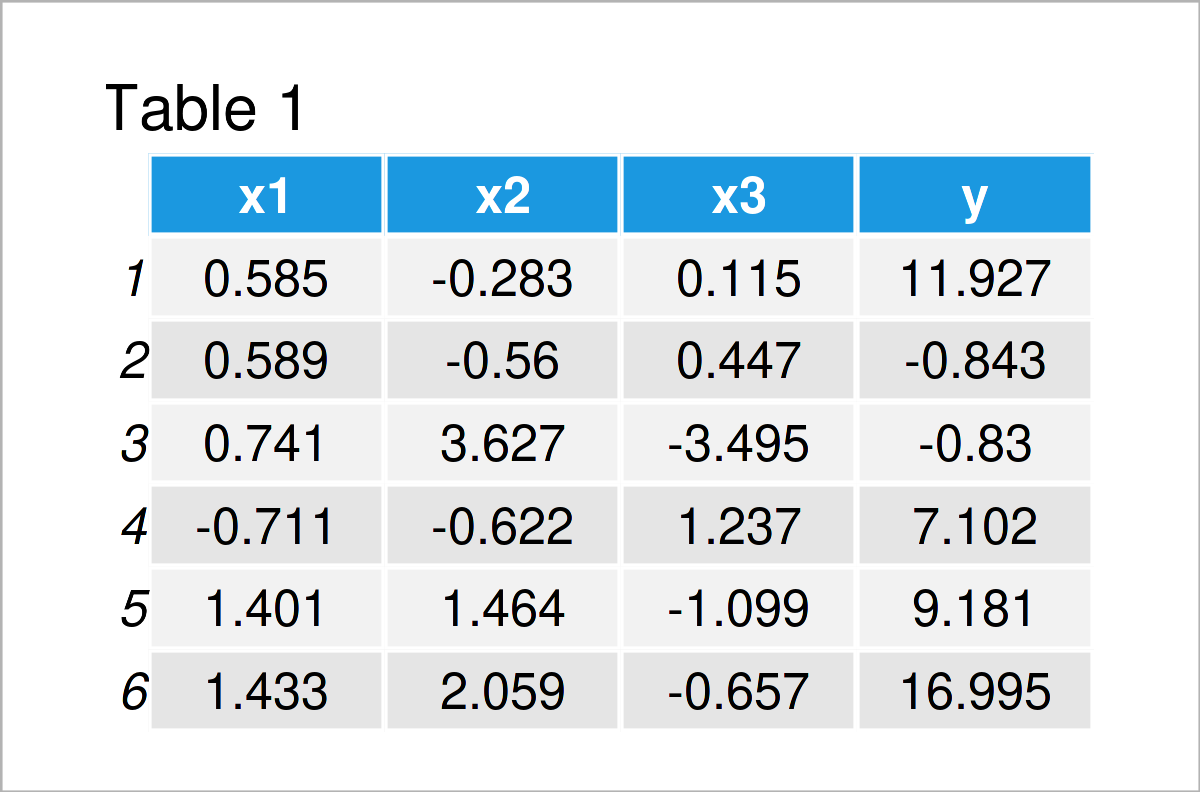# Extract Fitted Values from Regression Model in R (2 Examples)

In this tutorial you’ll learn how to get the fitted values of a linear regression model in R programming.

The tutorial contains this information:

Here’s how to do it!

## Construction of Example Data

The following data is used as basement for this R tutorial:

```set.seed(293675)                 # Create example data
x1 <- rnorm(100)
x2 <- rnorm(100) + x1
x3 <- rnorm(100) + 0.2 * x1 - 0.5 * x2
y <- rnorm(100, 10, 10) + x1 + x2 + x3
data <- data.frame(x1, x2, x3, y)Table 1 illustrates the RStudio console output and shows that our example data contains four columns. The variables x1, x2, and x3 will be used as predictors (independent variables) and the variable y as target variable (dependent variable).

Let’s estimate a linear regression model based on our example data:

```my_mod <- lm(y ~ ., data)        # Estimate linear regression model
summary(my_mod)                  # Summary of linear regression model
# Call:
# lm(formula = y ~ ., data = data)
#
# Residuals:
#      Min       1Q   Median       3Q      Max
# -25.6855  -6.4824  -0.0359   6.2732  22.5334
#
# Coefficients:
#             Estimate Std. Error t value Pr(>|t|)
# (Intercept)  10.1381     1.0170   9.968  < 2e-16 ***
# x1            0.6526     1.3744   0.475  0.63600
# x2            2.3580     1.0631   2.218  0.02891 *
# x3            2.8050     0.8534   3.287  0.00142 **
# ---
# Signif. codes:  0 ‘***’ 0.001 ‘**’ 0.01 ‘*’ 0.05 ‘.’ 0.1 ‘ ’ 1
#
# Residual standard error: 9.864 on 96 degrees of freedom
# Multiple R-squared:  0.1422,	Adjusted R-squared:  0.1154
# F-statistic: 5.303 on 3 and 96 DF,  p-value: 0.001999```

The previous output shows the output of our linear model. In the next examples, I’ll show how to extract the fitted values of this model.

## Example 1: Get Fitted Values of Linear Regression Model Using fitted() Function

This example demonstrates how to find the fitted values of a linear regression model using the fitted() function.

Have a look at the R syntax below:

```fit1 <- fitted(my_mod)           # Apply fitted function
#         1         2         3         4         5         6
# 10.173905 10.457139  9.370953 11.676833 11.420710 14.084482```

The previous output shows the first six fitted values (i.e. the head) corresponding to the first six observations in our data.

## Example 2: Get Fitted Values of Linear Regression Model Using predict() Function

In this section, I’ll show how to use the predict function instead of the fitted function to return the fitted values of our model.

In the present example, we simply have to use the predict function instead of the fitted function:

```fit2 <- predict(my_mod)          # Apply predict function
#         1         2         3         4         5         6
# 10.173905 10.457139  9.370953 11.676833 11.420710 14.084482```

As you can see, the result is the same as in Example 1.

Please note that this is not the case for all types of models. This is because the predict function by default returns predictions on the scale of the linear predictor.

For instance, in case of a binomial logit model the result of the fitted and predict functions would be different.

We could still use the predict function for such models, but we would have to specify the type argument to tell the predict function what kind of output we expect (e.g. predict(my_mod, type = “response”)).

For that reason, I usually prefer to use the fitted function to extract fitted values from a model in R.

## Video, Further Resources & Summary

Have a look at the following video on my YouTube channel. In the video, I’m showing the topics of this tutorial:

In addition to the video, you may want to have a look at the other articles on this homepage.

In this R programming tutorial you have learned how to return the fitted values of a linear regression model, and you have learned about the difference between the fitted and predict functions. Let me know in the comments section below, in case you have any further comments and/or questions. Furthermore, please subscribe to my email newsletter in order to receive updates on the newest articles.

Subscribe to the Statistics Globe Newsletter

•lihong
February 19, 2022 10:22 am

cool thanks

•February 19, 2022 5:40 pm

Thank you Lihong, glad you like the tutorial!

•wei wan
May 22, 2022 9:22 pm

Thank you !

•May 23, 2022 6:50 am

You are very welcome Wei Wan!

•Hanh
June 28, 2022 1:04 pm

Thanks for sharing. Can I use this for glm model?

•June 28, 2022 3:08 pm